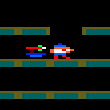### CPC Basic

Would you like to react to this message? Create an account in a few clicks or log in to continue.

# Double Buffering in CPC BASIC 3Out Bush

Posts : 11
Join date : 2019-12-02
I was able to create a Double Buffer example using Locomotive BASIC and a little Sprite Driver I created, but am having problems with it.

I've setup CPC BASIC 3, so it generates an Assembly file, I can then modify the Assembly file, so the Code is relocated to &8000, leaving the Screen at &4000 available, I can then Assemble using the incliuded Pasmo Assembler, but I use Winape which lets me Pause and Load the Object file on the Debugger screen.

Like my earlier version I was trying to have working with Locomotive BASIC, the problem occurs when I try to move the Ball up the screen, I thought that perhaps some adjustments I'd made in this version from the original Locomotive would get it working, but it's not quite as clear cut.

Code:
`100 DEFWORD a-z:GOSUB 2000110 MODE 0:BORDER 0:INK 0,3:INK 1,26:INK 2,21120 CALL &A02E:CLS:CALL &A028:CLS:CALL &A02E130 sc=2:x1=40:oy=720:ox=40140 i=720:i1=720:y1=720150 GOSUB 1310160 WHILE 1170  IF INKEY(2)=0 THEN IF y1<>1920 THEN GOSUB 1110180  IF INKEY(0)=0 THEN IF y1>0 THEN GOSUB 1140190  IF INKEY(1)=0 THEN IF x1<>76 THEN GOSUB 1170200  IF INKEY(8)=0 THEN IF x1>0 THEN GOSUB 1200210 WEND 220 END800 ' Move Down810 IF y1>&3FFF THEN i1=i+&50:i=i+&50:y1=i1820 GOSUB 1410:GOSUB 1510830 RETURN840 IF y1>&3FFF THEN i1=i+&50:i=i+&50:y1=i1850 GOSUB 1610:GOSUB 1310860 RETURN900 ' Move Up910 IF y1<2 THEN i2=i+&37B0:i=i-&50:y1=i2920 GOSUB 1410:GOSUB 1510930 RETURN940 IF y1<2 THEN i2=i+&37B0:i=i-&50:y1=i2950 GOSUB 1610:GOSUB 1310960 RETURN1000 ' Move Right1010 GOSUB 1410:GOSUB 15101020 RETURN1050 ' Move Left1060 GOSUB 1610:GOSUB 13101070 RETURN1100 ' Check Screen Status and Move accordingly1110 IF sc=1 THEN oy=z:y1=y1+&800:GOSUB 810:sc=2:RETURN1120 IF sc=2 THEN oy=z:y1=y1+&800:GOSUB 840:sc=1:RETURN1130 RETURN1140 IF sc=1 THEN oy=z:y1=y1-&800:GOSUB 910:sc=2:RETURN1150 IF sc=2 THEN oy=z:y1=y1-&800:GOSUB 940:sc=1:RETURN1160 RETURN1170 IF sc=1 THEN ox=w:x1=x1+1:GOSUB 1010:sc=2:CALL &BD19:RETURN1180 IF sc=2 THEN ox=w:x1=x1+1:GOSUB 1060:sc=1:CALL &BD19:RETURN1190 RETURN1200 IF sc=1 THEN ox=w:x1=x1-1:GOSUB 1010:sc=2:CALL &BD19:RETURN1210 IF sc=2 THEN ox=w:x1=x1-1:GOSUB 1060:sc=1:CALL &BD19:RETURN1220 RETURN1300 ' Draw screen to &40001310 s=&40001320 z=y11330 w=x11340 CALL &A000,&A036,(s+z)+w1350 CALL &A0281360 RETURN1400 ' Clear Screen at &C0001410 s=&C0001420 oz=oy1430 ow=ox1440 CALL &A000,&A056,(s+oz)+ow1450 RETURN1500 ' Draw screen at &C0001510 s=&C0001520 z=y11530 w=x11540 CALL &A000,&A036,(s+z)+w1550 CALL &A02E1560 RETURN1600 ' Clear Screeb at &40001610 s=&40001620 oz=oy1630 ow=ox1640 CALL &A000,&A056,(s+oz)+ow1650 RETURN2000 addr=&A0002010 FOR ln=1 TO 72020 READ a\$2030 FOR p=1 TO 31 STEP 22040 POKE addr,VAL("&"+MID\$(a\$,p,2))2050 addr=addr+12060 NEXT p:NEXT ln2070 RETURN2100 DATA DD6E02DD6603EBDD6E00DD66012234A02110 DATA 0608C506041A77132310FA2A34A0CD262120 DATA BC2234A0C110EBC93E40CD08BCC93EC02130 DATA CD08BCC900C0000000000040800000842140 DATA 480000844800008448000084480000402150 DATA 800000000000000000000000000000002170 DATA 00000000000000000000000000000000`

Current date/time is Thu Aug 18, 2022 7:19 pm